• +91 9971497814
• info@interviewmaterial.com

# RD Chapter 21- Surface Areas and Volume of a Sphere Ex-21.2 Interview Questions Answers

### Related Subjects

Question 1 :
Find the volume of a sphere whose radius is
(i) 2 cm
(ii) 3.5 cm
(iii) 10.5 cm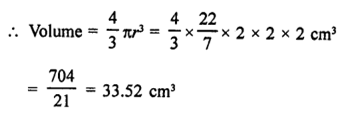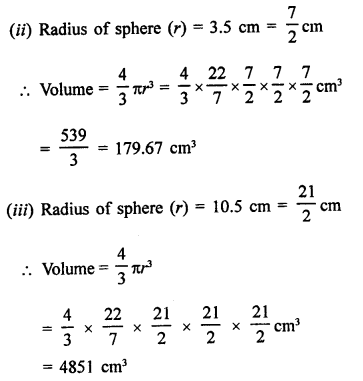Question 2 :
Find the volume of a sphere whose diameter is,
(i) 14 cm
(ii) 3.5 dm
(iii) 2.1 m

Answer 2 : (i) Diameter of a sphere = 14 cm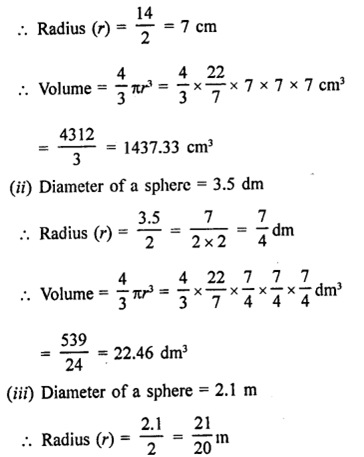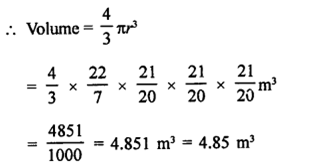Question 3 : A hemspherical tank has inner radius of 2.8 m. Find its capacity in litres.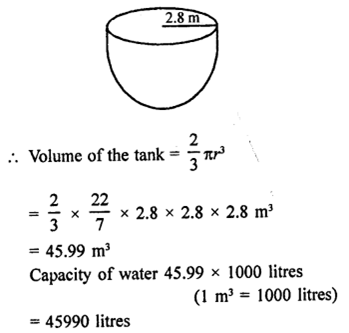Question 4 : A hemispherical bowl is made of steel 0.25 cm thick. The inside radius of the bowl is 5 cm. Find the volume of steel used in making the bowl.

Answer 4 : Thickness of steel = 0.25 cm = 1/4cm
Inside radius of the hemispherical bowl (r) = 5 cm
Outer radius (R) = 5 +0.25 = 5.25 cm
Volume of the steel used=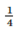π(R3 – r3)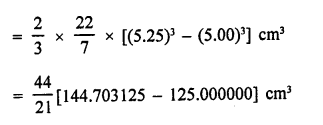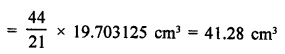Question 5 : How many bullets can be made out of a cube of lead,whose edge measures 22 cm, each bullet being 2 cm in diameter?

Answer 5 : Edge of cube (r) = 22 cm
Volume = a3 =(22)3 cm3
= 22 x 22 x 22 = 10648 cm3
Diameter of a bullet = 2 cm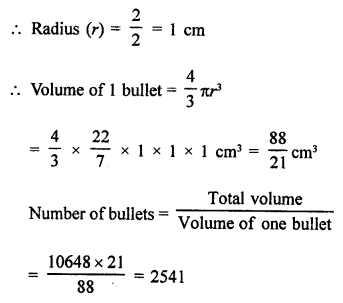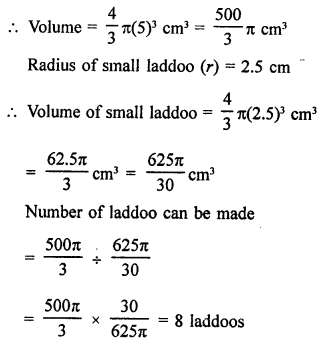Question 7 : A spherical ball of lead 3 cm in diameter is melted and recast into three spherical balls. It the diameters of two balls be 3/2 cm and 2 cm, find the diameter of the third ball.

Answer 7 : Diameter of a spherical ball of lead = 3 cm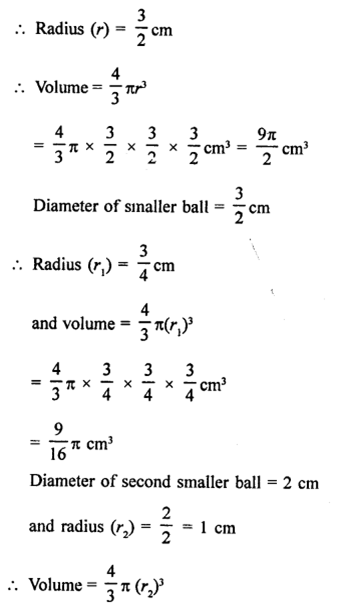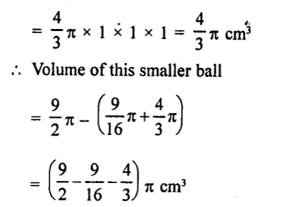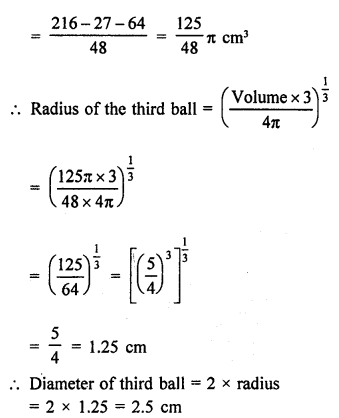Question 8 : A sphere of radius 5 cm is immersed in water filled in a cylinder, the level of water rises 5/3 cm. Find the radius of the cylinder.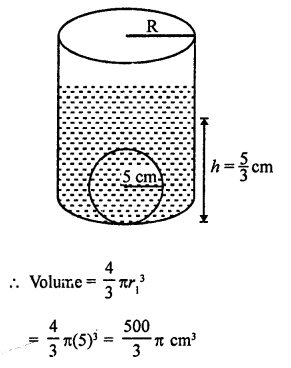Level of water rises in the cylinder after immersing the sphere in it
∴ Height of water level = 5/3 cm
Let r be radius of the cylinder, then Volume of water = Volume of the sphere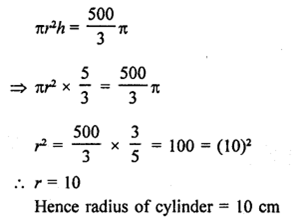Question 9 : If the radius of a sphere is doubled, what is the ratio of the volumes of the first sphere to that of the second sphere?

Let r2 be the radius of the given sphere
then volume =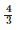πr3
By doubling the radius the radius of the new sphere = 2r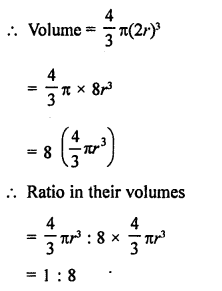Question 10 : A vessel in the form of a hemispherical bowl is full of water. Its contents are emptied in a right circular cylinder. The internal radii of the bowl and the cylinder are 3.5 cm and 7 cm respectively. Find the height to which the water will rise in the cylinder.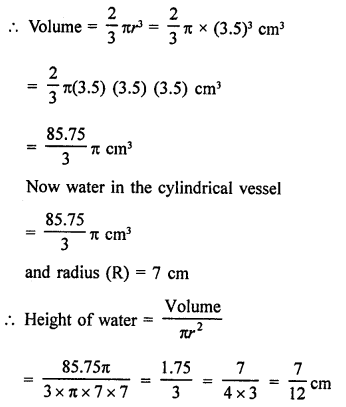Todays Deals### RD Chapter 21- Surface Areas and Volume of a Sphere Ex-21.2 Contributorskrishan

Name:
Email:

# Latest News# 9000 interview questions in different categories